# What is PID Controller? | Most Important Information

Hi Friends! Hope you all are doing well! You heard many times about PID Controller.

Today in this article we are going to see in-depth about PID Control. Implementation of our logic in PID Control in the PLC program.

So, Let’s not waste more time and get a deep dive into this…

# What is PID Control?

Nowadays, the PID controller is widely used in almost all types of industries. PID control gives a user, functionality for a particular function to work automatically as per user input.

There are dedicated PID controllers available in the market. As well as PLC also has an inbuilt PID controller block, which users can program as per their requirements.

Some of the most common types of industrial examples in which PID is used,

• Temperature control
• Pressure control
• Flow rate control
• Motors speed control
• Weigh-feeder rate control, etc.

There are many more complex PID control examples also,

• Furnace temperature control
• Ratio control of Pressure and Flow, etc

Regarding all that to know more about What is PID control?, we have to first know about the definition of PID.

## What is PID?

PID stands for Proportional, Integral, and Derivative. These three functions are combined in such a way that they can control the process as per the defined parameter.

Let’s take a simple example to understand, how PID Controller works?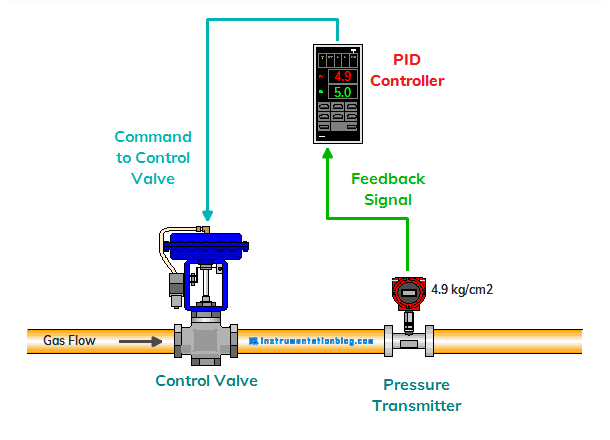In this example, we are maintaining the pressure of a gas by giving a command to the control valve. Here we have used a PID Controller in which we are giving a set value of how much pressure we want to maintain?

Instead of using a PID Controller, we can also use the inbuilt PID Control block of the PLC and programmed as per user requirement.

## Proportional Controller(P-Control)

The proportional controller generates an output which is directly proportional to the error. Now here the error is the difference between the set value and process value. This value of error will be multiplied with the proportional gain that determines the response of the output.

m = (Kp * e) + b

where,

• Kp = Proportional gain
• e = Error
• b = Bias
• m = Controller output

If we set gain too high, then there may be a chance of output becomes oscillating and unstable. If we set gain too low, then the controller takes too much time to set the process or maybe a chance that the controller will not respond to the process.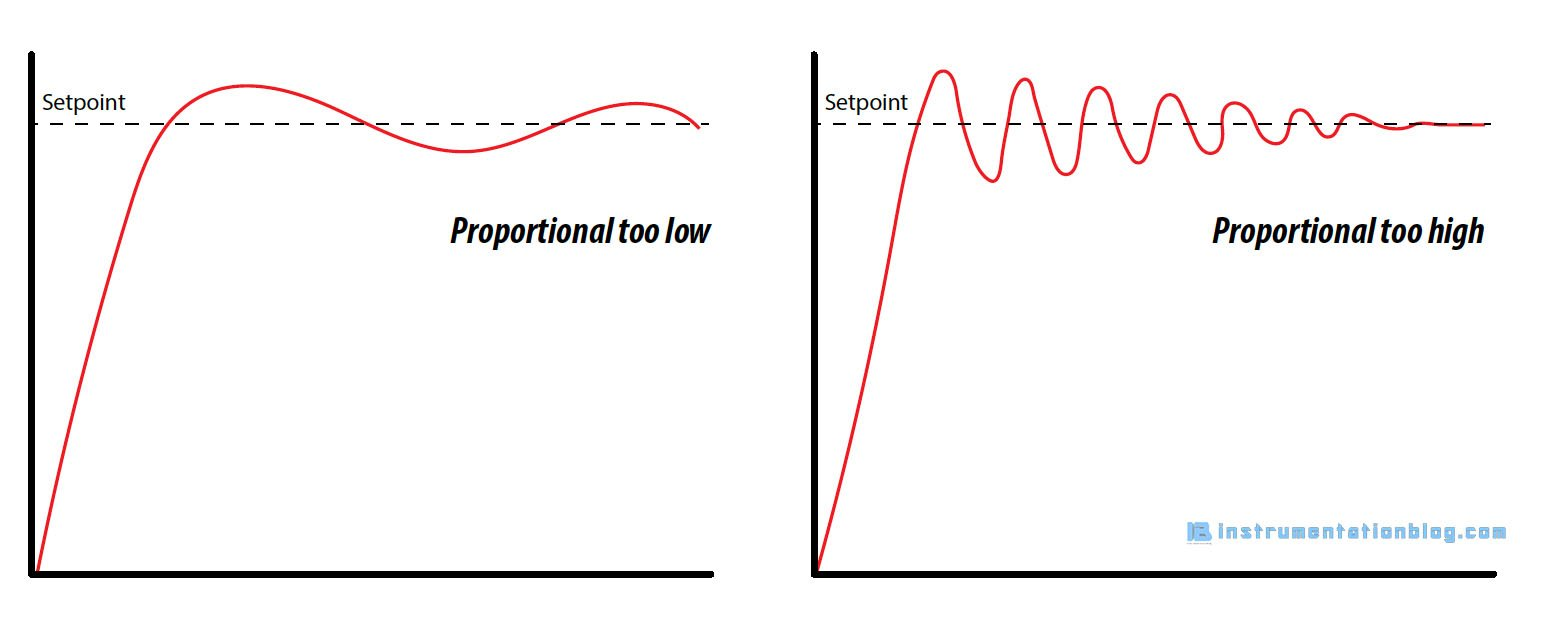There is a problem with a proportional-only controller. A well-tuned proportional only controller has also a sustained error in the graph. This sustained error is also called offset in proportional-only control. This error can be reduced adding Integral and Derivative control.

## Proportional-Integral Controller(PI-Control)

As we have seen that there is an offset is always present in the Proportional-only control. The Integral control will always increment and decrement the output value to reduce the error.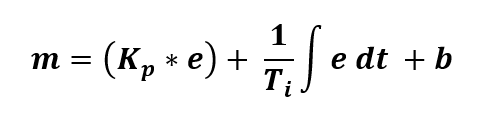where,

• Kp = Proportional gain
• e = Error
• Ti = Integral time constant
• t = Time
• b = Bias
• m = Controller output

In this formula, we can see the integration part ” ∫ e dt “. Please don’t confuse in this part, this integration part tells us that the controller adds up multiple products of error over a tiny period. Ti is the integration time which is given by the user.

To see how this works? Let’s consider Proportional + Integral actions. As we have seen that there is an offset that is continuous in the proportional only controller. To eliminate this offset Integral mode is used. Once the offset is present, an integral part now comes into action. The integral mode continuously adds up the multiplied value of error over some time, this value will be added in the output until the offset will become smaller near to zero.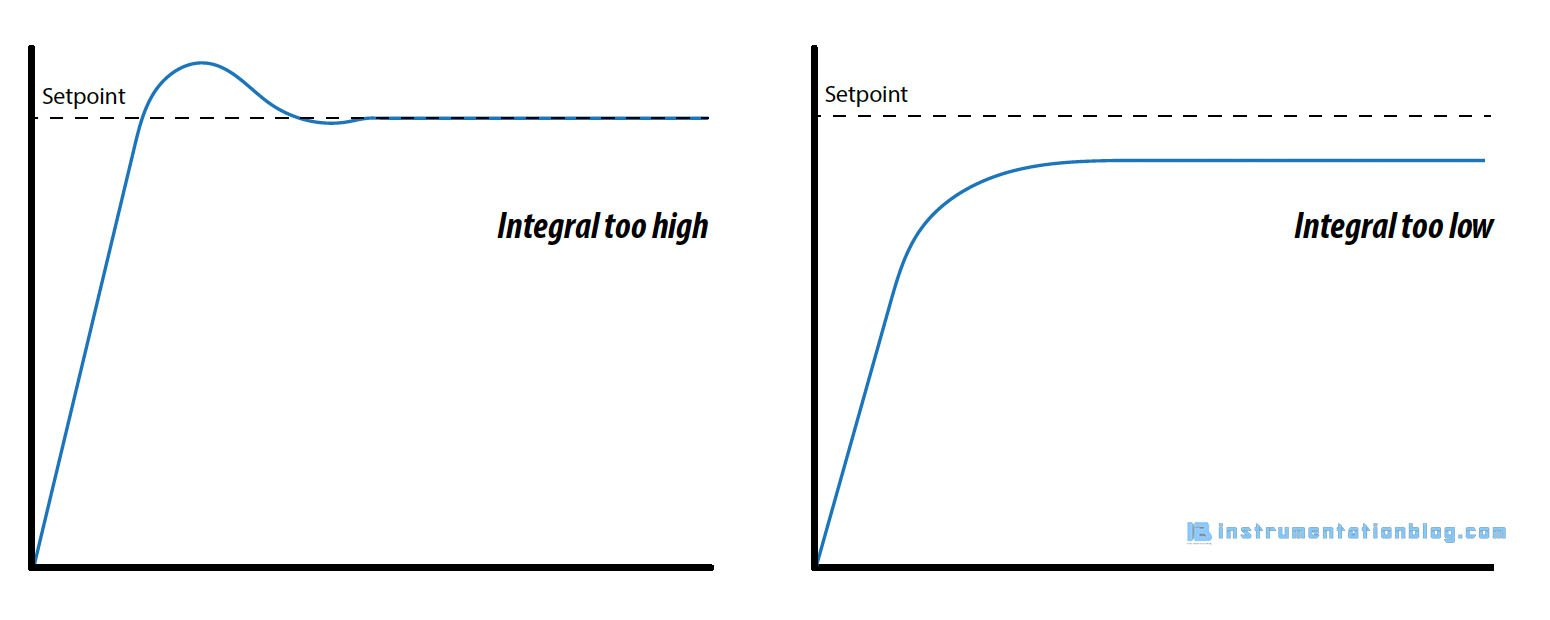For the larger value of Ti, the response of the controller output will become slow and for the smaller value of Ti, the response of the controller output will become fast.

## Proportional-Integral-Derivative Controller(PID-Control)

In the end, there is a “D” term which is Derivative control. Derivative finds the rate of change of error with respect to time.

As we all know that Derivative of the function is the inverse operation of integration. Integration calculates the accumulated value of the variable’s product with respect to time, On the other hand, Derivative calculates the ratio of the variable’s change with the unit change of time.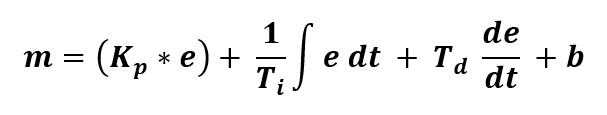where,

• Kp = Proportional gain
• e = Error
• Ti = Integral time constant
• t = Time
• Td = Derivative time constant
• b = Bias
• m = Controller output

The derivative controller always looks for the rate of change of process variable per unit change of time and takes action proportional to that. That is the way it limits the speed of the change of process variable.

With a larger value of derivative time, it may be a chance of output becomes oscillating and become unstable.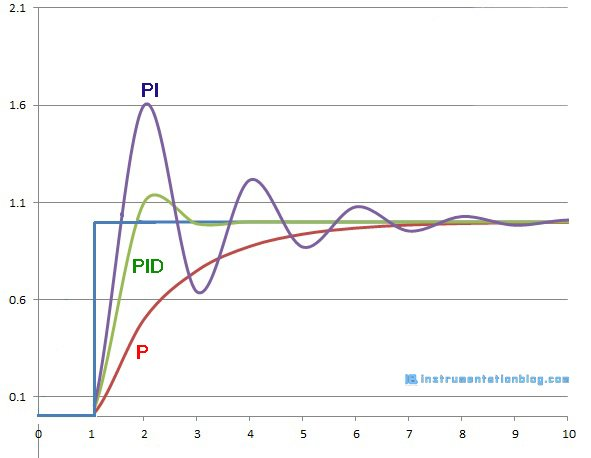I hope you got a basic idea about the PID controller and how PID Control works?  You can also read about the closed-loop vs open-loop control. If you want more articles like these then please comment down below. Thanks!

Programmable Logic Controller (PLC) related more articles ⇒